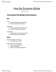# ECON 1B03 Lecture Notes - Lecture 15: Economic Surplus, Economic Equilibrium, Demand Curve

16 views4 pages
School
Department
Course
Professorlilachyena933 and 37504 others unlocked46
Verified Note
46 documents

## Document Summary

Workshop externalities, welfare, public goods, price controls. Set supply equal to demand: 300-p = 4p , so p = equilibrium price. Sub this in to either equation to get equilibrium quantity = 240 units. Sketch: first solve for the intercepts set equations equal to 0, Consumer surplus area below demand curve and above equilibrium price. Difference between what consumers are willing to pay and the total amount they actually pay ie consumer benefit. Benefit to suppliers who sell at price higher than they are willing to produce for. Area above supply curve but below equilibrium price. Ps= ((60-0)(240))= : government freezes price level at . Plug the new price into the equations to find the value of the shortage. Shortage = qd qs = 255 -180 = 75 units. The new consumer surplus is between the price freeze and the new. Plug the new qs into demand surplus ---- 180 = 300-p, p = 120.

## Get access

\$8 USD/m\$10 USD/m
Billed \$96 USD annuallyHomework Help
Study Guides
Textbook Solutions
Class Notes
Textbook Notes
Booster Class
Class+
\$8 USD/m
Billed \$96 USD annuallyHomework Help
Study Guides
Textbook Solutions
Class Notes
Textbook Notes
Booster Class